Related Articles

# Class 10 NCERT Solutions- Chapter 13 Surface Areas And Volumes – Exercise 13.3

• Difficulty Level : Medium
• Last Updated : 10 Mar, 2021

### Question 1. A metallic sphere of radius 4.2 cm is melted and recast into the shape of a cylinder of radius 6 cm. Find the height of the cylinder.

Solution: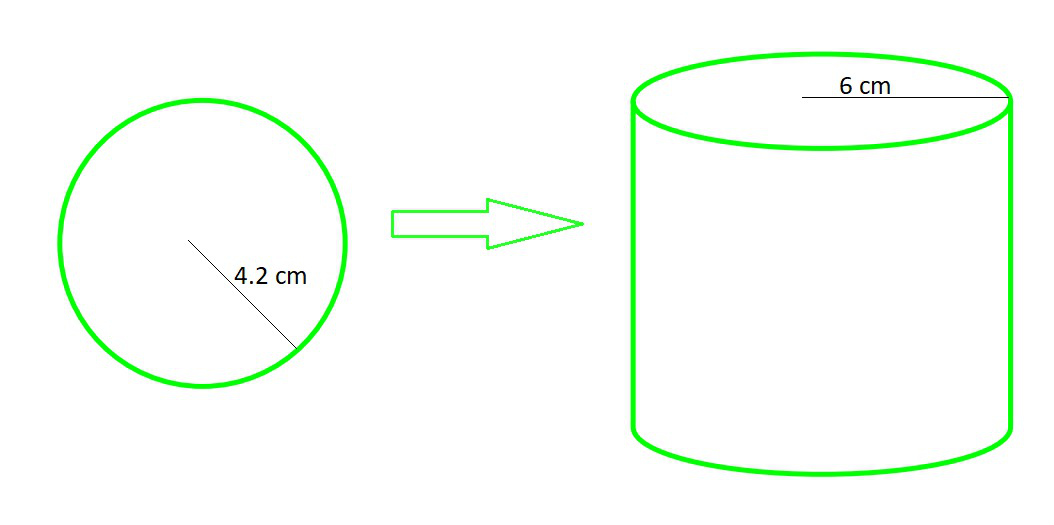Radius of sphere (R)= 4.2 cm

Radius of cylinder (r)= 6 cm

In recasting process the volume will be same, so

Volume of cylinder = Volume of sphere

πr2h =πR3

π(6)2h =π(4.2)3                                                 (cancel π from both side)

36h =(4.2 × 4.2 × 4.2)

h =(cm)

h = 1.4 × 1.4 × 1.4 (cm)

h = 2.74 cm

### Question 2. Metallic spheres of radii 6 cm, 8 cm and 10 cm, respectively, are melted to form a single solid sphere. Find the radius of the resulting sphere.

Solution: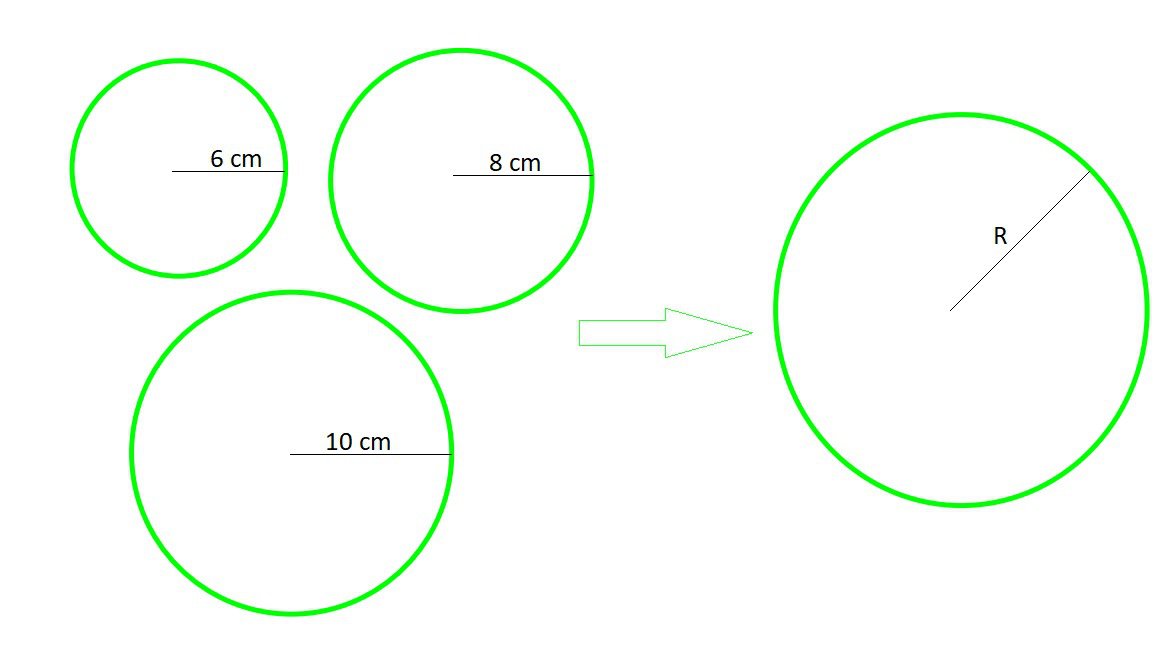Radius of sphere 1 (r1)= 6 cm

Radius of sphere 2 (r2)= 8 cm

Radius of sphere 3 (r3)= 10 cm

Let Radius of resulting sphere = R

In recasting process the volume will be same, so

Volume of Resulting sphere = Volume of sphere 1 + Volume of sphere 2 + Volume of sphere 3π(R)3π(r1)3π(r2)3 +π(r3)3                           (cancelπ from both side)

R3 = (r1)3 + (r2)3 + (r3)3

R3 = (6)3 + (8)3 + (10)3

R3 = 216 + 512 + 1000

R3 = 1728

R = (1728) 1/3

R = 12 cm

### Question 3. A 20 m deep well with diameter 7 m is dug and the earth from digging is evenly spread out to form a platform 22 m by 14 m. Find the height of the platform.

Solution: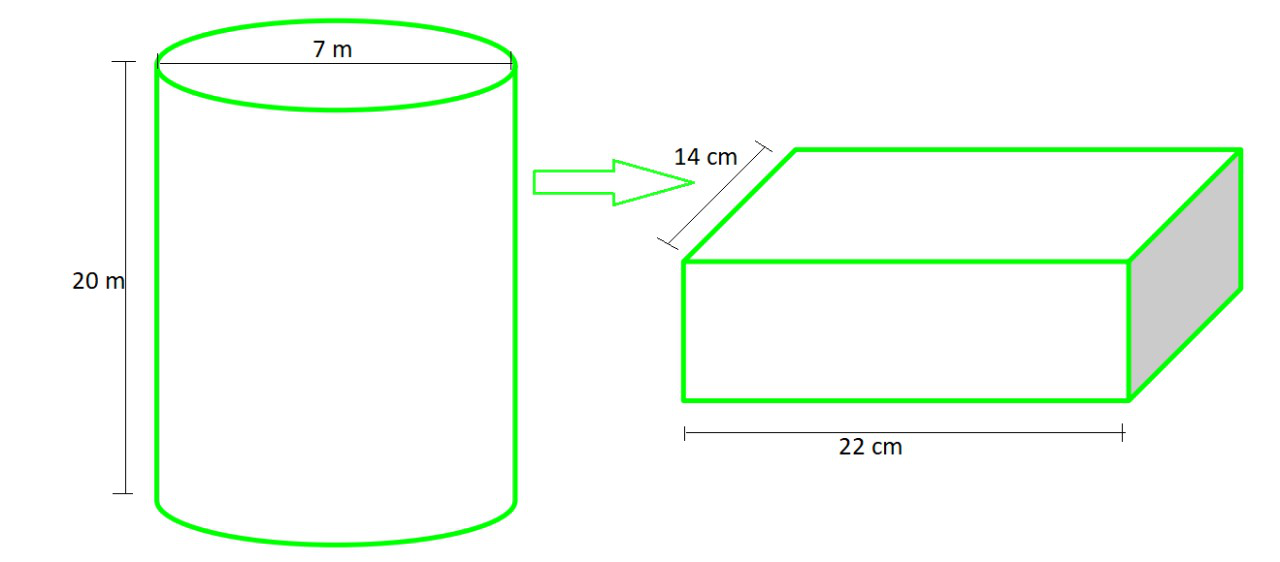So basically here, the digging of cylindrical shape is changed to cuboidal shape

Given values,

Diameter of cylinder = 7 m

Radius of cylinder (r)=m

Height of cylinder (H)= 20 m

Length of Cuboid (l) = 22 m

Breadth of Cuboid (b) =14 m

Let Height of Cuboid = h

In this process the volume will be same, so

Volume of Cuboid = Volume of Cylinder

l × b × h = πr2H

22 × 14 × h = π ×× 20

h =m                                  (taking π =)

h =m

h = 2.5 m

### Question 4. A well of diameter 3 m is dug 14 m deep. The earth taken out of it has been spread evenly all around it in the shape of a circular ring of width 4 m to form an embankment. Find the height of the embankment.

Solution: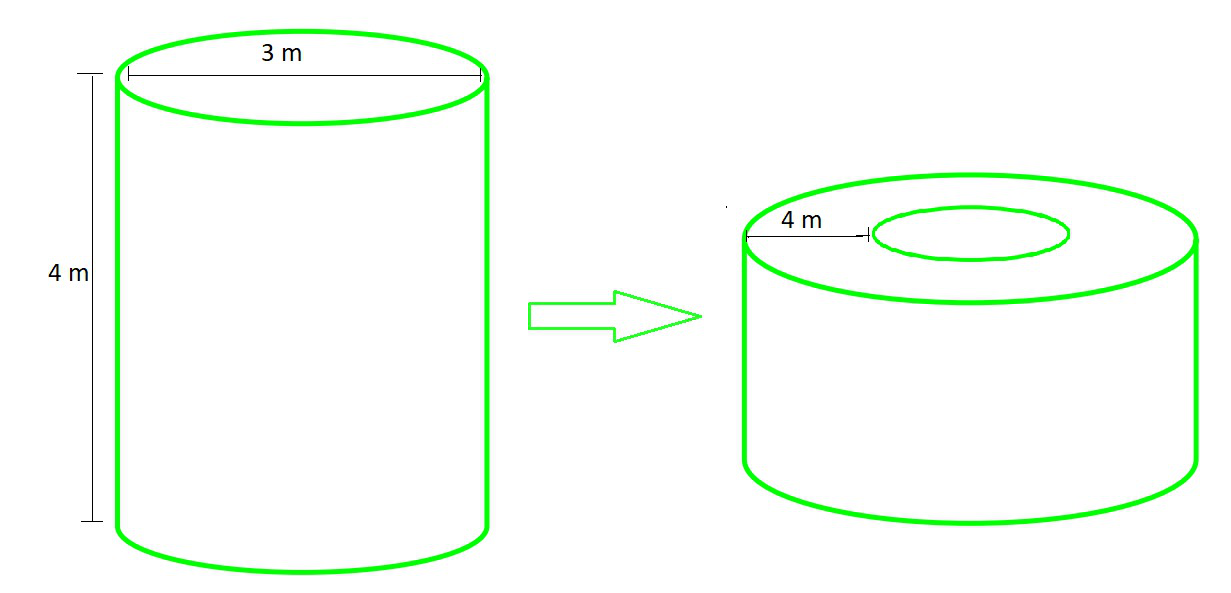So basically here, the digging of cylindrical shape is changed to another Hollow cylindrical shape

Given values,

Diameter of cylinder = 3 m

Radius of cylinder (r) =m

Height of cylinder (h) = 14 m

Width of embankment = 4 m

Outer Radius of embankment R1= radius of cylinder + width = 3/2 + 4 =m

Inner Radius of embankment R2= radius of cylinder =m

Height of embankment = H

In this process the volume will be same, so

Volume of Embankment = Volume of Cylinder

(πR12H) – (πR22H) = πr2h

(R12 R22)H = ()2× 14                                                               (cancel π from both side)

H =H =H =H =H =H =m

H = 1.125 m

### Question 5. A container shaped like a right circular cylinder having diameter 12 cm and height 15 cm is full of ice cream. The ice cream is to be filled into cones of height 12 cm and diameter 6 cm, having a hemispherical shape on the top. Find the number of such cones which can be filled with ice cream.

Solution: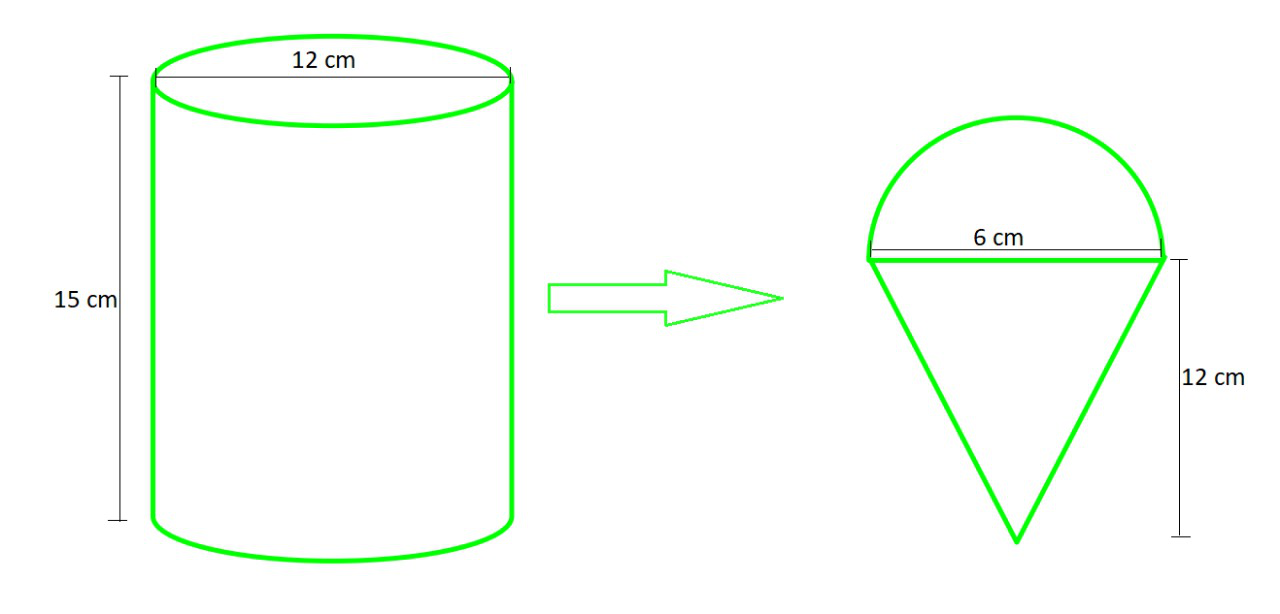Given values,

Radius of cylinder (r) = 6 cm

Height of cylinder (h) = 15 cm

Radius of each cone (R) = 3 cm

Height of each cone (H) = 12 cm

Let n be the total number of ice creams

In this process the volume will be same, so

n × (Volume of each Cone + Volume of each hemisphere) = Volume of Cylinder

n × (πR2H +πR3) = πr2h

n =(cancel π from both side)

n =n =n =n = 10

Hence, 10 numbers of cones filled with ice-cream.

### Question 6. How many silver coins, 1.75 cm in diameter and of thickness 2 mm, must be melted to form a cuboid of dimensions 5.5 cm × 10 cm × 3.5 cm?

Solution: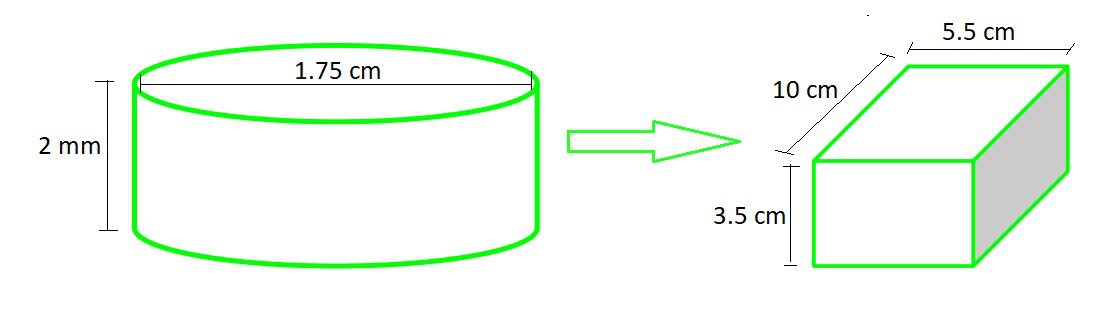Given values,

Radius of cylindrical coin (r) =Height of cylindrical coin (H) = 2 mm =cm

Length of Cuboid (l) = 5.5 cm

Breadth of Cuboid (b) =10 cm

Height of Cuboid (h)= 3.5 cm

Let n be the total number of coins

In this process the volume will be same, so

n × (Volume of each Coin) = Volume of Cylinder

n × (πR2H) = l × b × h

n × π × ()2×= 5.5 × 10 × 3.5

n =(taking π =)

n =n = 400

Hence, 400 silver coins must be melted to form this cuboid.

### Question 7. A cylindrical bucket, 32 cm high and with radius of base 18 cm, is filled with sand. This bucket is emptied on the ground and a conical heap of sand is formed. If the height of the conical heap is 24 cm, find the radius and slant height of the heap.

Solution:

So basically here, the cylindrical shape is changed to conical shape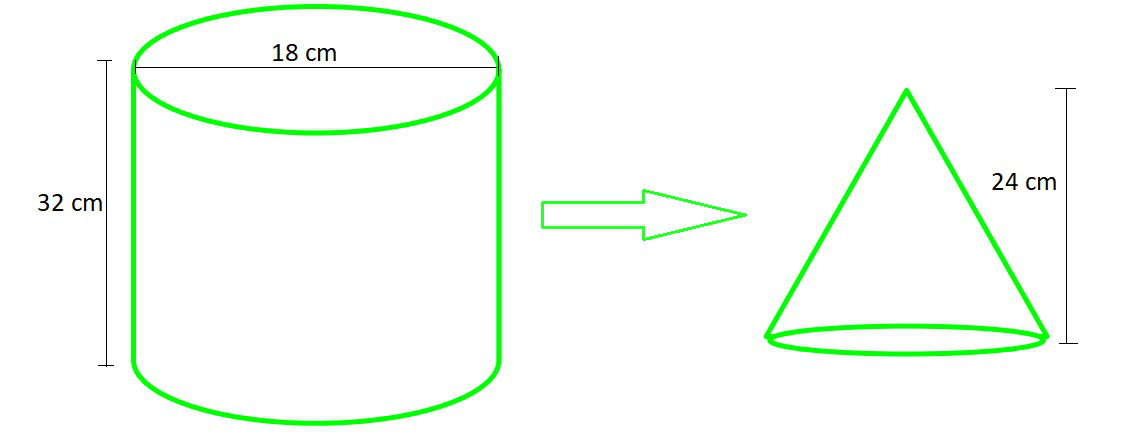Given values,

Radius of cylinder (r) = 18 cm

Height of cylinder (h) = 32 cm

Height of cone (H) = 24 cm

Let Radius of cone = R

In this process the volume will be same, so

Volume of Cone = Volume of CylinderπR2H = πr2hR2 × (24) = 182 × 32                                           (cancel π from both side)

R2R = √(18 × 18 × 4)

R = 36 cm

Slant height (l) = √H2 + R2

l = √(242 + 362)

l = √(12×2)2 + (12×3)2

l =12 √(4+9)

l = 12 √13 cm

### Question 8. Water in a canal, 6 m wide and 1.5 m deep, is flowing with a speed of 10 km/h. How much area will it irrigate in 30 minutes, if 8 cm of standing water is needed?

Solution:

Given values,

Width of canal (w) = 6 m

Depth of canal (h) = 1.5 m

Speed of canal = 10 km/hr = (10,000 m/hr)

For 1hr (60 mins), we can take length (l) as = 10,000 m and

Volume in 1hr = (l × w × h)

= (10,000 × 6 × 1.5) m3

= 90,000 m3

So for 30 mins volume will be =m3

= 45,000 m3

Area irrigated in 30 minutes will be as:

Area × length of standing = Volume of canal in 30mins

Area =Area = 562500 m2

### Question 9. A farmer connects a pipe of internal diameter 20 cm from a canal into a cylindrical tank in her field, which is 10 m in diameter and 2 m deep. If water flows through the pipe at the rate of 3 km/h, in how much time will the tank be filled?

Solution:

Given values,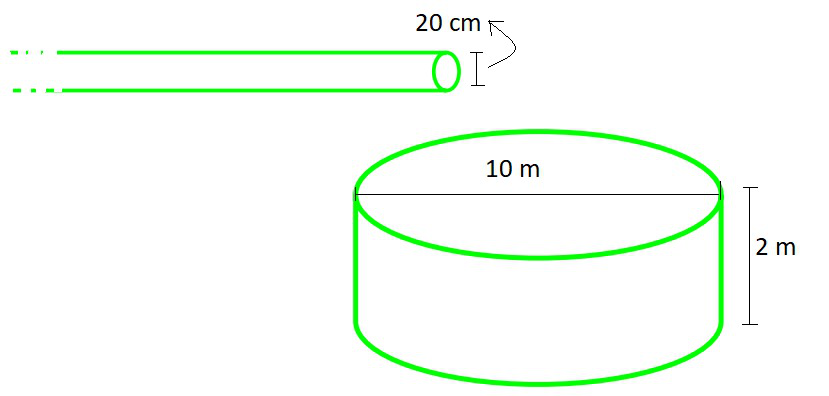Radius of Pipe (R) = 10 cm =m

Radius of Cylindrical tank (r) = 5 m

Depth of Cylindrical tank (h) = 2 m

Speed of water flows in pipe = 3 km/hr = (3,000 m/hr)

Let Time to fill cylindrical tank = t

For 1hr (60 mins), we can take height of Pipe (H) as = 3,000 m and

Volume in 1hr = (πR2H)

= (π × ()2 × 3,000) m3

= 30π m3

In this process the volume will be same, so

t (in hr) × (Volume of Pipe in 1hr) = Volume of Cylindrical Tank

t × (30π) = πr2h

t × (30π) = π × 52 × 2

t =t =hr

t =× 60 mins

t = 100 minutes

Hence, 100 minutes will be taken to fill the tank.

Attention reader! All those who say programming isn’t for kids, just haven’t met the right mentors yet. Join the  Demo Class for First Step to Coding Coursespecifically designed for students of class 8 to 12.

The students will get to learn more about the world of programming in these free classes which will definitely help them in making a wise career choice in the future.

My Personal Notes arrow_drop_up